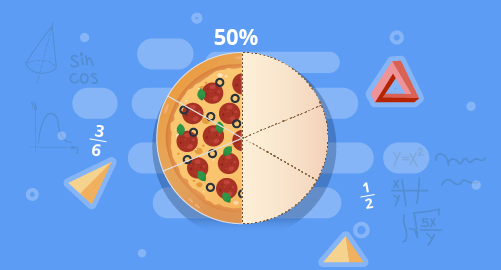#### Need Help?

Get in touch with usSAT/ACT

# Percentages (Fundamentals)

Maths

Find the percent of a quantity as rate per 100. Write percents in the form of fractions or decimals. Solve problems involving finding the whole, given a part and the percent.

##### Course Duration
•1 Weeks6 Sessions
•50 mins per session
##### Tutor## What you'll learn

• 1

Represent and find the percent of a whole.

• 2

Write equivalent value as fractions, decimal, or percents.

• 3

Write percents that are greater than 100 or less than 1.

• 4

Estimate the percent of a number using equivalent fractions, rounding, or compatible numbers.

• 5

Solve problems involving percents.

• 6

Find the whole amount when given a part and the percent.

## Skills you'll learnProblem solving